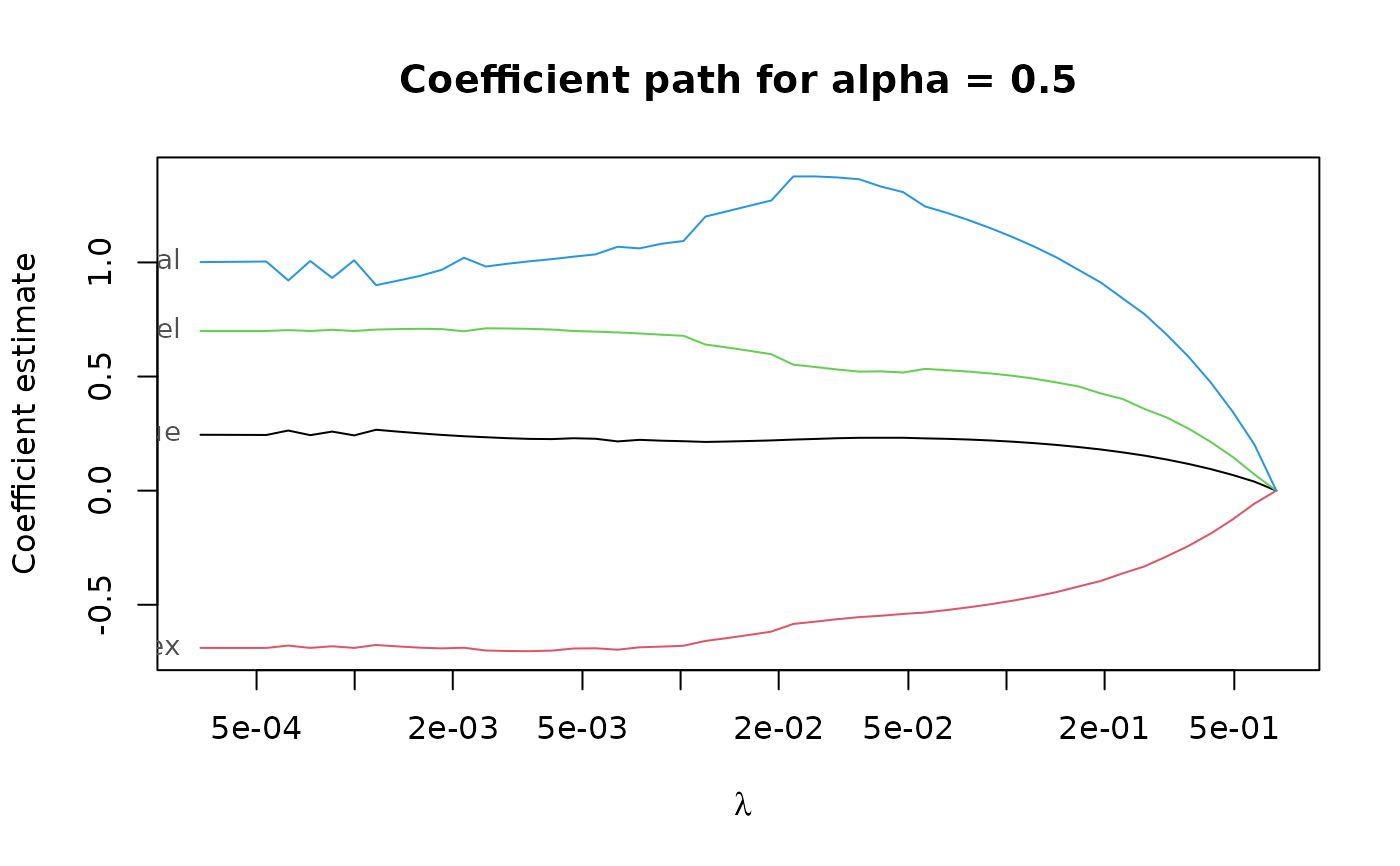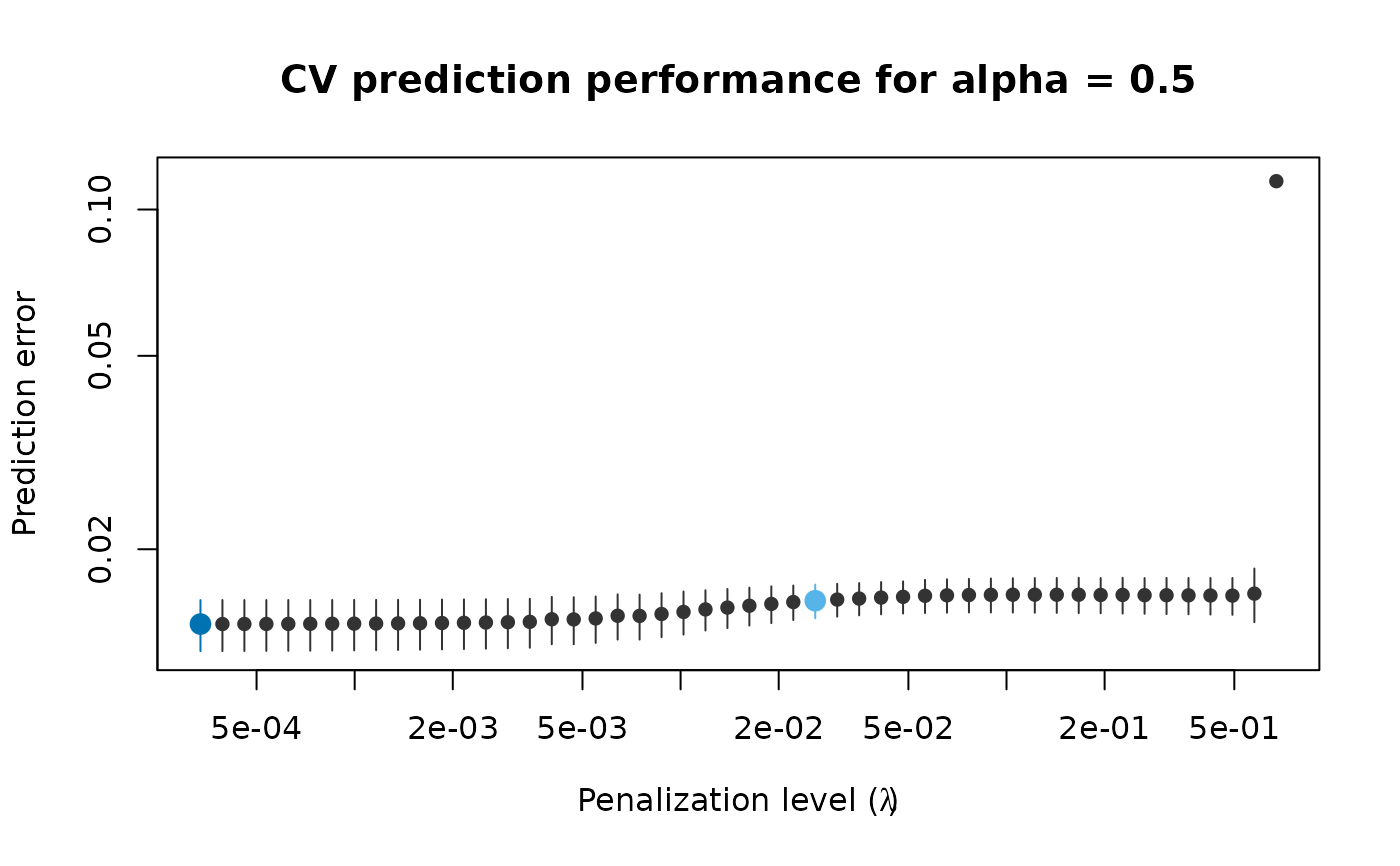Plot the cross-validation performance or the coefficient path for fitted penalized elastic net S- or LS-estimates of regression.

# S3 method for pense_cvfit
plot(x, what = c("cv", "coef.path"), alpha = NULL, se_mult = 1, ...)

x fitted estimates with cross-validation information. plot either the CV performance or the coefficient path. If what = "cv", only CV performance for fits with matching alpha are plotted. In case alpha is missing or NULL, all fits in x are plotted. If what = "coef.path", plot the coefficient path for the fit with the given hyper-parameter value or, in case alpha is missing, for the first value in x$alpha. if plotting CV performance, multiplier of the estimated SE. currently ignored. ## See also Other functions for plotting and printing: plot.pense_fit(), prediction_performance(), summary.pense_cvfit() ## Examples # Compute the PENSE regularization path for Freeny's revenue data # (see ?freeny) data(freeny) x <- as.matrix(freeny[ , 2:5]) regpath <- pense(x, freeny$y, alpha = 0.5)
plot(regpath)# Extract the coefficients at a certain penalization level
coef(regpath, lambda = regpath$lambda[][]) #> (Intercept) lag.quarterly.revenue price.index #> -6.6475338 0.2411667 -0.6985229 #> income.level market.potential #> 0.7098337 0.9619783 # What penalization level leads to good prediction performance? set.seed(123) cv_results <- pense_cv(x, freeny$y, alpha = 0.5,
cv_repl = 2, cv_k = 4)
plot(cv_results, se_mult = 1)# Extract the coefficients at the penalization level with
# smallest prediction error ...
coef(cv_results)
#>           (Intercept) lag.quarterly.revenue           price.index
#>            -8.5228825             0.2072828            -0.6946405
#>          income.level      market.potential
#>             0.6778202             1.1430756 # ... or at the penalization level with prediction error
# statistically indistinguishable from the minimum.
coef(cv_results, lambda = '1-se')
#>           (Intercept) lag.quarterly.revenue           price.index
#>            -8.9377554             0.2066104            -0.6851005
#>          income.level      market.potential
#>             0.6654687             1.1777421